# Evaluation Metrics for Classification Problems | Machine Learning

In the last article, I have talked about Evaluation Metrics for Regression, and In this article, I am going to talk about Evaluation metrics for Classification problems.

1. Accuracy
2. Precision
3. Recall
4. F1-Score

# Classification Problems:

In Classification problems, we try to predict and to identifying which of a set of categories a new observation belongs to, For Example; assigning a given email to the “spam” or “non-spam” class.

Before getting into the evaluation metrics, we have first to know about Confusion Matrix, which is a specific table layout that allows visualization of the performance of an algorithm and also to evaluate the accuracy of a classification problem.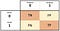Confusion Matrix

Each row of the matrix represents the instances in a predicted class, while each column represents the instances in an actual class (or vice versa).

0 is the negative class.
1 is the positive class.
TP: or True Positives, means the number of cases where the actual class was 1 and also the predicted class was 1.
TN: or True Negatives, means the number of cases where the actual class was 0 and also the predicted class was 0.
FP: or False Positives, means the number of cases where the predicted class was positive (1) and the actual class was negative (0)
FN: or False Negatives, means the number of cases where the predicted class was negative(0) and the actual class was positive(1)

I know, In the first impression, It seems very complicated but once you test with some real examples, you will realize that it was not a big deal, and here is an example that can help you to understand what I am trying to say; https://en.wikipedia.org/wiki/Confusion_matrix#Example

It is very important to understand the Confusion Matrix, because, we are going to need it when computing evaluation metrics,

Let’s consider this example: https://en.wikipedia.org/wiki/Confusion_matrix#Example, in which we have: cats belong to class 1 (positive class) and dogs belong to class 0 (negative class)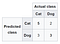Confusion Matric for the problem

# Accuracy:

we calculate the Accuracy, by dividing the total number of true predictions (TP + TN) by the total number of all the cases (TP + TN + FP + FN)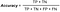Accuracy formula

In our example;
the accuracy = (5 +3 ) / (5+ 3 + 2 + 3) = 8/13 = 0.61 = 61%,
this result tells us that your model has classified 60% of the data correctly.

# Precision: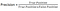Precision formula

and as you may notice in this metric, we focus just on the positive class or side.
In our example, the precision is going to be:

precision = 5 / (5 + 2) = 5/7 = 0.71.

Of course, you will ask, why do we need this precision while we have accuracy?
Good question, and to understand the difference and the importance of each metric, you have to work on different problems and cases, because, you will find out in some cases calculating accuracy is not good enough to determine if your model is doing a great job, and there are other cases where the recall metric is the better one.

# Recall: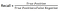Recall formula

in recall metric, we divide the true positives (TP = Actual positives and predicted positives 1) by the sum of the true positives and the false negatives (FN = Actual positives and predicted negatives)
In our example:
recall = 5 / (5+3) = 0.62

# F1-score: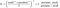F1-Score Formula

This is another metric, in which, we use precision and recall values.

In our example:
F1 = (2*0.71*0.62)/(0.71+0.62) = 0.66.

# Conclusion:

For classification problems, Accuracy is not always the best metric to evaluate your model, recall and precision can be better in some cases especially in imbalanced classification tasks (Credit Card fraud detection for example)

In the next article, I am going to give some examples where precision and recall can perform better than calculating accuracy, and you will see that accuracy does not provide a useful assessment on several crucial problems.

If you have any questions or clarifications do not hesitate to contact me, Thanks!

Computer Science student and passionate

## More from Idriss Jairi

Computer Science student and passionate